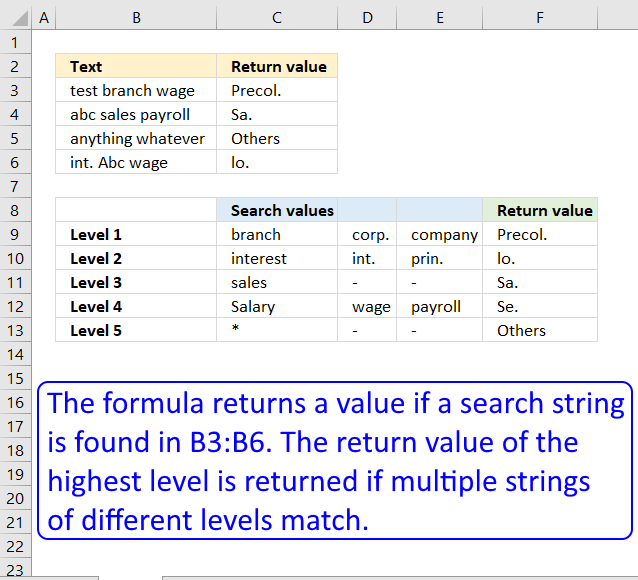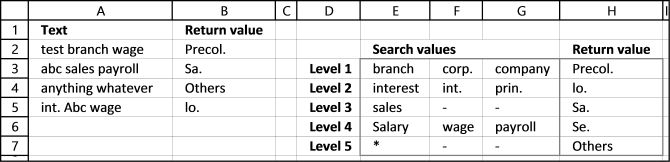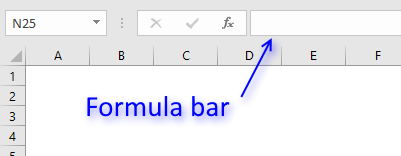Author: Oscar Cronquist Article last updated on February 01, 2019Minh Hung asks:

Hello Mr Oscar
I have the matter to create a megaformula to categorize my list. For short example:A1: Cash in deposit (Branch A t/t)
A2: Borrowed from Corp. A
A3: Interest payment
A4: Int.panalty pmt
A5: Prin. Pmt
A6: Salary Pmt on April
A7: Sales abroad
A8: Branch C t/t
A9: Transferred from Company AA
A10: Mortgages to DD ltd
A11: Sal. Pmt on Mayand
at B1 cell, I create a formula as follows:=IF(COUNT(SEARCH({"branch","corp.", "company"},A1))>0,"Precol.", IF(COUNT(SEARCH({"interest","int.", "prin."},A1))>0,"lo.",IF(COUNT(SEARCH("sales", A1))>0,"Sa.",IF(COUNT(SEARCH({"sal.","Salary", "wage","payroll"},A1))>0,"Se.","Others"))))But, my formula is too long and too many parentheses. I want to shorten this formula or replace by another. But how? Could you please to solve my question?Thank you very much.Hung

#### Answer:

By examining your formula it seems that you have different levels with search words. The word found with the lowest level should be returned, leave the remaining.Example 1, cell A2 contains "test branch wage". "branch" is found on level 1 (cell E3) and "wage" on level 4 (cell F6). However "branch" is on the lowest level of the two so Precol. (cell H3) is returned in cell B2.

Example 2, cell A3 contains "abc sales payroll". "sales" is a search string found on level 3 and "payroll" is on level 4. Level 3 is the lowest level so "Sa." (cell H5) is returned in cell B3.

Example 3, cell A4 contains"anything whatever" and no search value is found except the asterisk (*) on level 5.  Text string "Others" (cell H7) is returned in cell B4.

Array formula in cell B2:

=INDEX(\$H\$3:\$H\$7, MIN(IF(ISNUMBER(SEARCH(\$E\$3:\$G\$7, A2)), MATCH(ROW(\$E\$3:\$G\$7), ROW(\$E\$3:\$G\$7)), "")))

#### How to enter an array formula

1. Select cell B2
2. Type the formula above in formula bar3. Press and hold CTRL + SHIFT key
4. Press Enter

If you did above steps correctly excel automatically adds a beginning and ending curly bracket {array_formula} to the formula. Don't enter these characters yourself.

#### How does the array formula in cell B2 work?

You can easily follow along as I explain this array formula. Select cell B2 and go to "Formulas" on the ribbon, click "Evaluate Formula" button. Click on "Evaluate" to go to next step.

Step 1 - Search for multiple text strings simultaneously

The SEARCH function returns the number of the character at which a specific character or text string is found. However, we are only interested if the string is found or not, this function is exactly what we need.

We are doing something strange with the SEARCH function below, we are not only using one string but multiple strings at once. That is why you see a cell range in the first argument.

SEARCH(\$E\$3:\$G\$7,A2)

becomes

SEARCH({"branch","corp.","company";"interest","int.","prin.";"sales","-","-";"Salary","wage","payroll";"*","-","-"},"test branch wage")

and returns

{6, #VALUE!, #VALUE!; #VALUE!, #VALUE!, #VALUE!;#VALUE!, #VALUE!,#VALUE!; #VALUE!,13,#VALUE!; 1,#VALUE!, #VALUE!}

The SEARCH function returns an array with the same size as the cell range used in the first argument, see above.

\$E\$3:\$G\$7 is a cell range containing multiple columns and rows. An array with multiple columns and rows uses commas and semicolons as delimiting characters.

A comma is used as a delimiting character to separate values column by column and a semi colon is used to separate values row by row.

\$E\$3:\$G\$7 has three columns, E, F and G. The returning array above contains three values separated by two commas and then a semicolon, so the array has also three columns. The same with the number of rows in cell range \$E\$3:\$G\$7 and rows in array.

Step 2 - Look for numbers in array

A number indicates that the text string is found, an error #VALUE tells you that no search string is found. The ISNUMBER function converts numbers to TRUE and all other values including errors to FALSE.

ISNUMBER(SEARCH(\$E\$3:\$G\$7,A2))

becomes

ISNUMBER({6,#VALUE!,#VALUE!;#VALUE!, #VALUE!,#VALUE!;#VALUE!,#VALUE!,#VALUE!; #VALUE!,13,#VALUE!;1,#VALUE!,#VALUE!})

and returns {TRUE, FALSE, FALSE;FALSE, FALSE, FALSE;FALSE, FALSE, FALSE;FALSE, TRUE, FALSE;TRUE, FALSE, FALSE}

Step 3 - If text string is found return corresponding row number

IF(ISNUMBER(SEARCH(\$E\$3:\$G\$7, A2)), MATCH(ROW(\$E\$3:\$G\$7), ROW(\$E\$3:\$G\$7)),"")

becomes

IF({TRUE, FALSE, FALSE;FALSE, FALSE, FALSE;FALSE, FALSE, FALSE;FALSE, TRUE, FALSE;TRUE, FALSE, FALSE}, MATCH(ROW(\$E\$3:\$G\$7), ROW(\$E\$3:\$G\$7)), "")

becomes

IF({TRUE, FALSE, FALSE;FALSE, FALSE, FALSE;FALSE, FALSE, FALSE;FALSE, TRUE, FALSE;TRUE, FALSE, FALSE}, {1; 2; 3; 4; 5}, "")

and returns

{1,"","";"","","";"","","";"",4,"";5,"",""}

Step 4 - Extract smallest row number

MIN(IF(ISNUMBER(SEARCH(\$E\$3:\$G\$7, A2)), MATCH(ROW(\$E\$3:\$G\$7), ROW(\$E\$3:\$G\$7)), ""))

becomes

MIN({1,"","";"","","";"","","";"",4,"";5,"",""})

and returns 1.

Step 5 - Return value using row number

INDEX(\$H\$3:\$H\$7, MIN(IF(ISNUMBER(SEARCH(\$E\$3:\$G\$7, A2)), MATCH(ROW(\$E\$3:\$G\$7), ROW(\$E\$3:\$G\$7)), "")))

becomes

INDEX(\$H\$3:\$H\$7, 1)

becomes

INDEX({"Precol.";"lo.";"Sa.";"Se.";"Others"}, 1)

and returns Precol. in cell B2.

#### Download excel *.xlsx file

Nested Search.xlsx

#### Functions in this post

SEARCH(find_text,within_text, [start_num])
Returns the number of the character at which a specific character or text string is found reading left to rigt (not case-sensitive)

IF(logical_test, [value_if_true], [value_if_false])
Checks whether a condition is met, and returns one value if TRUE, and another value if FALSE

MATCH(lookup_value, lookup_array, [match_type])
Returns the relative position of an item in an array that matches a specified value

INDEX(array,row_num,[column_num])
Returns a value or reference of the cell at the intersection of a particular row and column, in a given range

ROW(reference)
Returns the rownumber of a reference